# 8th Grade - The Pythagorean Theorem

## Introduction

• Pythagorean Theorem is one of the most useful theorems in Geometry and Trigonometry.
• It has many applications in science and measurement involving right triangles.
• Theorem: In a right triangle, the square of the hypotenuse is equal to the sum of the squares of the legs.
• To illustrate, we have: ${\mathbit{c}}^{\mathbf{2}}\mathbf{=}{\mathbit{a}}^{\mathbf{2}}\mathbf{+}{\mathbit{b}}^{\mathbf{2}}$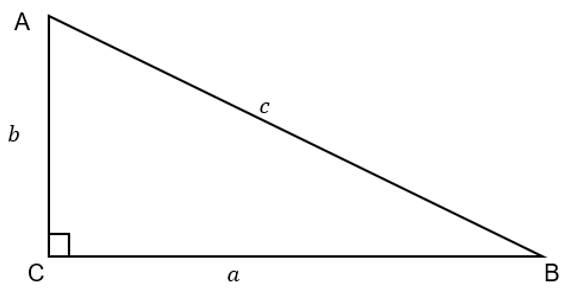• Converse: If in a right triangle, the square of the length of one side is equal to the sum of the square of the lengths of the other two sides, then the triangle is a right triangle, and the right angle is opposite the longest side.
• If $\mathbf{△}$ABC satisfies ${\mathbit{c}}^{\mathbf{2}}\mathbf{=}{\mathbit{a}}^{\mathbf{2}}\mathbf{+}{\mathbit{b}}^{\mathbf{2}}$, then $\mathbf{△}\mathbit{A}\mathbit{B}\mathbit{C}$ is a right triangle.

Relations:

• If ${\mathbit{c}}^{\mathbf{2}}\mathbf{=}{\mathbit{a}}^{\mathbf{2}}\mathbf{+}{\mathbit{b}}^{\mathbf{2}}$, then  are sides of a right triangle.
• If ${\mathbit{c}}^{\mathbf{2}}\mathbf{>}{\mathbit{a}}^{\mathbf{2}}\mathbf{+}{\mathbit{b}}^{\mathbf{2}}$, then  are sides of an obtuse triangle.
• If ${\mathbit{c}}^{\mathbf{2}}\mathbf{<}{\mathbit{a}}^{\mathbf{2}}\mathbf{+}{\mathbit{b}}^{\mathbf{2}}$, then  are sides of an acute triangle.

## The Pythagorean Theorem Proof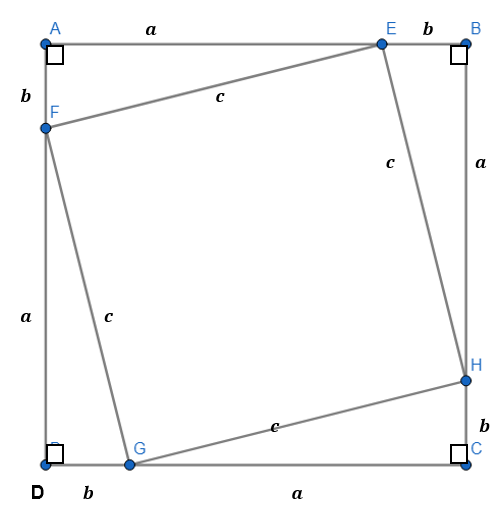• There are numerous ways to prove the Pythagorean Theorem. The one that is shown here is something about using the concept of the  Area of the square. You can try this by making some cut-outs on a piece of paper.
• The side of the square shown above is .
• An inscribed square is constructed so that four congruent right angles (by LL Theorem) will be formed with $\mathbit{c}$ as side of the inscribed square and hypotenuse of each of the four right triangles.
• Using Area Addition Postulate, the Area of the Larger Square = Area of the Inscribed Square + Area of Four Right Triangles. This is described as:

${\left(a+b\right)}^{\mathbf{2}}\mathbf{=}{\mathbit{c}}^{\mathbf{2}}\mathbf{+}\mathbf{4}\left(\frac{1}{2}ab\right)\mathbf{\to }{\mathbit{a}}^{\mathbf{2}}\mathbf{+}\mathbf{2}\mathbit{a}\mathbit{b}\mathbf{+}{\mathbit{b}}^{\mathbf{2}}\mathbf{=}{\mathbit{c}}^{\mathbf{2}}\mathbf{+}\mathbf{2}\mathbit{a}\mathbit{b}$

• By the Subtraction Property of Equality and the Symmetric Property of Equality, we get ${\mathbit{c}}^{\mathbf{2}}\mathbf{=}{\mathbit{a}}^{\mathbf{2}}\mathbf{+}{\mathbit{b}}^{\mathbf{2}}$.
• The obtained equation is called the Pythagorean Formula.
• The numbers  are called the Pythagorean Triples.

## Solved Examples

Example 1. A tree is broken 6 meters above the level ground. The top strikes the ground 8 meters from the foot, while the other end of the broken part remains attached to the trunk. Find the length of the broken piece from the trunk to the ground.

Diagram: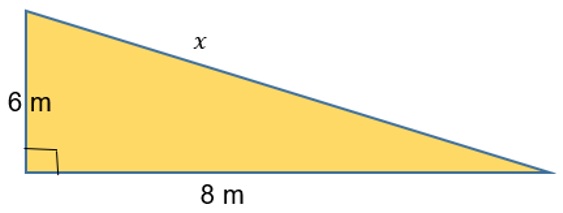The broken part is the hypotenuse which is x meters.

Example 2. Which of the following sides are sides of a right triangle? A. , B. , C.

Apply the Converse of the Pythagorean Theorem

Let $\mathbit{c}$ be the longest side of each triangle.

Only satisfies the equation ${\mathbit{c}}^{\mathbf{2}}\mathbf{=}{\mathbit{a}}^{\mathbf{2}}\mathbf{+}{\mathbit{b}}^{\mathbf{2}}$.

## Pythagorean Triples

• In a right triangle, we can prove that for any positive integer $\mathbit{n}$, the positive numbers  satisfy the Pythagorean equation.
• Assume as the length of the legs, and ${\mathbit{n}}^{\mathbf{2}}\mathbf{+}\mathbf{1}$ as the hypotenuse.
• For $\mathbf{1}\mathbf{<}\mathbit{n}\mathbf{\le }\mathbf{5}$, we can form 4 possible combinations of the sides of a right triangle.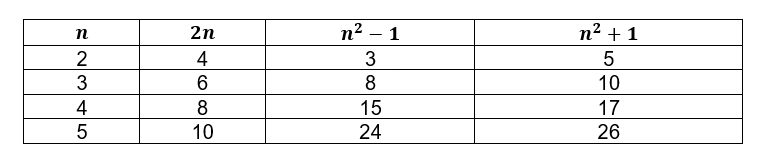• Another interesting way to form the Pythagorean Triples is by using the relation of two positive integers where $\mathbit{m}\mathbf{>}\mathbit{n}$.
• This topic is more expounded in Number Theory wherein the Pythagorean equation ${\mathbit{c}}^{\mathbf{2}}\mathbf{=}{\mathbit{a}}^{\mathbf{2}}\mathbf{+}{\mathbit{b}}^{\mathbf{2}}$ is a second-degree Diophantine equation wherein all primitive solutions are given by the relationships
• The integers  are said to have opposite parity if and only if one of them is odd and the other is even.
• They are called the same parity if both of them are odd or both of them are even.
• Two integers  are coprime, that is $\mathbit{g}\mathbit{c}\mathbit{d}\left(m,n\right)\mathbf{=}\mathbf{1}$.
• We can also create a sample table of Pythagorean Triples by using the two integers .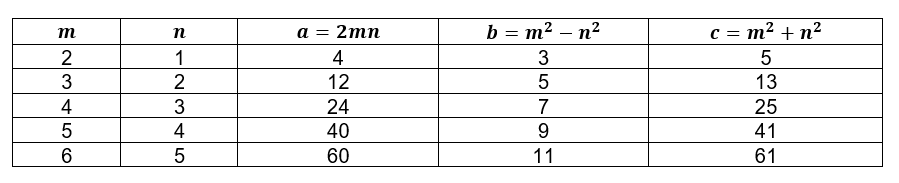## Cheat Sheet

• In the Pythagorean Theorem, .
• In Pythagorean Theorem: If $\mathbf{△}\mathbit{A}\mathbit{B}\mathbit{C}$ is a right triangle, then ${\mathbit{c}}^{\mathbf{2}}\mathbf{=}{\mathbit{a}}^{\mathbf{2}}\mathbf{+}{\mathbit{b}}^{\mathbf{2}}$.
• In Converse of the Pythagorean Theorem: If ${\mathbit{c}}^{\mathbf{2}}\mathbf{=}{\mathbit{a}}^{\mathbf{2}}\mathbf{+}{\mathbit{b}}^{\mathbf{2}}$, then $\mathbf{△}\mathbit{A}\mathbit{B}\mathbit{C}$ is a right triangle.
• The converse of the Pythagorean Theorem is helpful in determining the type of triangle according to angle measure.
• In $△ABC$, if $\mathbit{C}$ is $\mathbf{90}\mathbf{°}$, then it is opposite the hypotenuse denoted by $\mathbit{c}$

## Blunder Areas

• In computing for the length of sides or distances using the Pythagorean Theorem, always take the positive value when taking square roots since negative values are geometrically impossible.
• The essence of the formula ${c}^{2}={a}^{2}+{b}^{2}$ as the Pythagorean Theorem is not realized unless it is specified that $c$ is the length of the hypotenuse and the legs are
• The sides of the right triangle are not close to the variables . Any letter symbol, big letters or small letters, even Greek alphabets, are applicable as long as the concept of the theorem is used.
• In solving for the side lengths of a right triangle where each side is represented by an algebraic expression and the resulting equation is quadratic, always take the positive root. Always double-check whether the obtained value will satisfy the Pythagorean Equation or not.